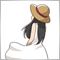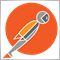# Remove duplicates635

Hi,

I want to get all Symbols in my present orderlist in an array. But if the symbol is already in the list, the loop must take the next one. I am stuck here. Maybe someone knows how

```string Pair[];
ArrayResize(Pair,1);
int size=1;

for(int i=0;i<=OrdersTotal();i++)  {
if (OrderMagicNumber() == sMagicNumber){
for (int y=0;y<ArraySize(Pair);y++)
{
if (OrderSymbol() == Pair[y])
break;
else
{
Pair[y]=OrderSymbol();
if (Pair !="")
ArrayResize(Pair,size+1);
}
}
}
}
}
```

So in the example there should be 3 Pairs: EURAUD,EURJPY,GBPJPY.

What do I do wrong?

Files:
Symbols.jpg 33 kb524

```string Pair;
int size=1;
bool flag;

for(int i=0;i<OrdersTotal();i++)
{
{
flag=false;

for(int y=0;y<ArraySize(Pair)-1;y++)
{
if(OrderSymbol()==Pair[y])
{
flag=true;
break;
}
}

if(!flag)
{
Pair[size-1]=OrderSymbol();
size++;
ArrayResize(Pair,size);
}
}
}```635

Thank you. This was a good advise. In the end it looks like this.

```int size=1,y;
bool flag;
string Pair;

for(int i=0;i<OrdersTotal();i++)  {
//Print("Size: " + ArraySize(Pair_Source));
flag=false;
if(OrderMagicNumber() == sMagicNumber || OrderMagicNumber() == sMagicNumber+1) {
for(y=0;y<ArraySize(Pair);y++) {
if(OrderSymbol()==Pair[y] && (OrderMagicNumber() != sMagicNumber || OrderMagicNumber() != sMagicNumber+1)) {
flag=true;
break;
}
}

if(!flag)      {
//Print("y: " + y + " || i: " + i + " || Symbol: " + OrderSymbol());
Pair[size-1]=OrderSymbol();
size++;
ArrayResize(Pair,size);
//Print(ArraySize(Pair));
}
}
}
}
ArrayResize(Pair,ArraySize(Pair)-1);```2392

```#include <arrays/arraystring.mqh>

void OnStart()
{
CArrayString symbols;
for (int i=0; OrderSelect(i, SELECT_BY_POS); i++)
if (symbols.SearchLinear(OrderSymbol()) < 0)
for (int i=0; i<symbols.Total(); i++)
Print(symbols[i]);
}```20210

1. Simplify your code by refactoring, (or use the CArrayString class.)

```template<typename T>int linearSearch(T item, const T& array[], int iEnd=-1, int iBeg=0){
if(iEnd == -1) iEnd = ArraySize(array);
for(; iBeg < iEnd; ++iBeg) if(item == array[iBeg]) break;
return iBeg;
}
template<typename T>int insertUnique(T item, T& array[], int iEnd=-1, int iBeg=0){
if(iEnd == -1) iEnd = ArraySize(array);
if(iEnd == linearSearch(item, array, iEnd, iBeg){
ArrayResize(array, iEnd+1); array[iEnd] = item; ++iEnd;
}
return iEnd;
}
int openSymbols(string& s[]){
for(int i=0;i<OrdersTotal();i++) if(
&& (OrderMagicNumber() == sMagicNumber || OrderMagicNumber() == sMagicNumber+1)
) insertUnique(OrderSymbol(), s);
return ArraySize(s);
}

string Pair[];
int nPair = openSymbols(Pair);
```

2. Florian Riedrich:
```if(OrderMagicNumber() == sMagicNumber || OrderMagicNumber() == sMagicNumber+1) {
for(y=0;y<ArraySize(Pair);y++) {
if(OrderSymbol()==Pair[y] && (OrderMagicNumber() != sMagicNumber || OrderMagicNumber() != sMagicNumber+1)) {
```
The second condition will always be true. If (m==1 or m==2) then (m!=1 or m!=2) If it's 1 then it's not 2 and if it's 2 then it's not 1.635